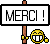[Livre] Foundations of Data Science

This book provides an introduction to the mathematical and algorithmic foundations of data science, including machine learning, high-dimensional geometry, and analysis of large networks. Topics include the counterintuitive nature of data in high dimensions, important linear algebraic techniques such as singular value decomposition, the theory of random walks and Markov chains, the fundamentals of and important algorithms for machine learning, algorithms and analysis for clustering, probabilistic models for large networks, representation learning including topic modelling and non-negative matrix factorization, wavelets and compressed sensing. Important probabilistic techniques are developed including the law of large numbers, tail inequalities, analysis of random projections, generalization guarantees in machine learning, and moment methods for analysis of phase transitions in large random graphs. Additionally, important structural and complexity measures are discussed such as matrix norms and VC-dimension. This book is suitable for both undergraduate and graduate courses in the design and analysis of algorithms for data.

[Lire la suite]